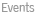backMini-Curso em "Overdispersion and Quasi-Likelihood for Discrete data"
 When applying generalized linear models to discrete data, we frequently meet overdispersion relative to the Poisson or binomial models. Such overdispersion can be explained by specific mixture models such as the negative binomial or the beta-binomial, or by making second-moment assumptions and use quasi-likelihood estimation. The quasi-likelihood method has a certain degree of robustness, as it depends on second-moment assumptions only, while being optimal among all linear estimating functions based on Pearson residuals. However, the lack of specific distributional assumptions makes simulation more difficult in the quasi-likelihood case. On the other hand, a specific mixture model, such as the negative binomial, can be fitted using maximum likelihood, which is fully efficient, given full distributional assumptions, but may also involve more heavy computations. However, the full distributional assumptions allow more straightforward simulation studies to be carried out. We propose the Neyman Type A distribution (a Poisson mixture of Poissons), fitted by quasi-likelihood, as a useful alternative to the negative binomial distribution for overdispersed discrete data. We shall also consider the more general Poisson-Tweedie mixture family, which includes both the negative binomial and the Neyman Type A as special cases. back© 2023 Universidade do Minho  - Legal Terms  - updated by CMATD.